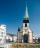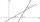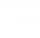# Climb

Road has climbing 1:27. How big is a angle corresponds to this climbing?

Result

α =  2.12 °

#### Solution:Leave us a comment of example and its solution (i.e. if it is still somewhat unclear...):

Showing 0 comments:Be the first to comment!#### To solve this example are needed these knowledge from mathematics:

See also our right triangle calculator. See also our trigonometric triangle calculator.

## Next similar examples:

1. RoadBetween cities A and B is route 13 km long of stúpanie average 7‰. Calculate the height difference of cities A and B.
2. SteepleSteeple seen from the road at an angle of 75°. When we zoom out to 25 meters, it is seen at an angle of 20°. What is high?
3. Height differenceWhat height difference overcome if we pass road 1 km long with a pitch21 per mille?
4. Trapezoid MOThe rectangular trapezoid ABCD with right angle at point B, |AC| = 12, |CD| = 8, diagonals are perpendicular to each other. Calculate the perimeter and area of ​​the trapezoid.
5. Ratio iso triangleThe ratio of the sides of an isosceles triangle is 7:6:7 Find the base angle to the nearest answer correct to 3 significant figure.
6. Two forcesTwo forces with magnitudes of 25 and 30 pounds act on an object at angles of 10° and 100° respectively. Find the direction and magnitude of the resultant force. Round to two decimal places in all intermediate steps and in your final answer.
7. Church towerArchdeacon church in Usti nad Labem has diverted tower by 186 cm. The tower is 65 m high. Calculate the angle by which the tower is tilted. Result write in degree's minutes.
8. Trigonometric functionsIn right triangle is: ? Determine the value of s and c: ? ?
9. RightDetermine angles of the right triangle with the hypotenuse c and legs a, b, if: ?
10. IS triangleCalculate interior angles of the isosceles triangle with base 38 cm and legs 26 cm long.
11. MastMast has 17 m long shadow on a slope rising from the mast foot in the direction of the shadow angle at angle 9.3°. Determine the height of the mast, if the sun above the horizon is at angle 44°30'.
12. RectangleCalculate the length of the side GN and diagonal QN of rectangle QGNH when given: |HN| = 25 cm and angle ∠ QGH = 28 degrees.
13. Right triangleCalculate the missing side b and interior angles, perimeter and area of ​​a right triangle if a=10 cm and hypotenuse c = 16 cm.
14. Angle between linesCalculate the angle between these two lines: ? ?
15. Regular 5-gonCalculate area of the regular pentagon with side 7 cm.
16. Q-ExamIf tg α = 0.9, Calculating sin α, cos α, cotg α .
17. Sphere in coneA sphere of radius 3 cm desribe cone with minimum volume. Determine cone dimensions.